Electron. J. Differential Equations, Vol. 2019 (2019), No. 24, pp. 1-22.

### Multiplicity and concentration of positive solutions for fractional nonlinear Schrodinger equations with critical growth Xudong Shang, Jihui Zhang

Abstract:
In this article we consider the multiplicity and concentration behavior of positive solutions for the fractional nonlinear Schrodinger equation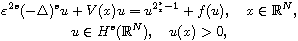where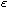is a positive parameter,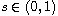, N >2s and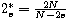is the fractional critical exponent, and f is a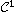function satisfying suitable assumptions. We assume that the potential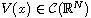satisfies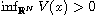, and that there exits k points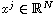such that for each j=1,...,k,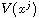are strictly global minimum. By using the variational method, we show that there are at least k positive solutions for a small>0. Moreover, we establish the concentration property of solutions astends to zero.

Submitted March 10, 2017. Published February 12, 2019.
Math Subject Classifications: 35A15, 58E05.
Key Words: Fractional Schrodinger equations; multiplicity of solutions; critical growth; variational method.

Show me the PDF file (346 KB), TEX file for this article.Xudong Shang School of Mathematics Nanjing Normal University Taizhou College 225300, Jiangsu, China email: xudong-shang@163.com Jihui Zhang Jiangsu Key Laboratory for NSLSCS School of Mathematical Sciences Nanjing Normal University Nanjing 210023, China email: zhangjihui@njnu.edu.cn